﻿ William Crawley-Boevey - Bielefeld University
BIREP - Representations of finite dimensional algebras at Bielefeld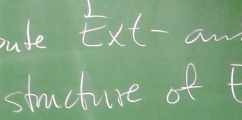# Prof. Dr. William (=Bill) Crawley-Boevey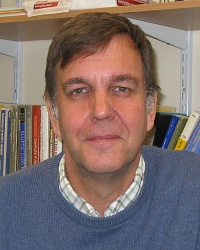### Alexander von Humboldt Professor

Research group: BIREP

E-Mail: wcrawley
Telephone: +49 (0)521 106 5033
Office: V5-221 (Now back from temporary office).

 Address: Fakultät für Mathematik Universität Bielefeld Postfach 100131 33501 Bielefeld Germany

# Research position

Advertised here in english and here in german. Closing date 10.11.2023.

# CRC

I am a participant in Collaborative Research Centre 358 on "Integral Structures in Geometry and Representation Theory". I am a principal investigator on projects A6, C2, C3.

# Miscellaneous

### Research Students

• Roberto Vila Freyer completed an Oxford D. Phil. on the topic 'Biserial algebras' in 1994.
• Nicola Richmond completed a Leeds Ph. D. on the topic 'The geometry of modules over finite dimensional algebras' in 1999.
• Andrew Hubery completed a Leeds Ph. D. on the topic 'Representations of quivers respecting a quiver automorphism and a theorem of Kac' in 2002.
• Peter Shaw completed a Leeds Ph. D. on the topic 'Generalisations of Preprojective algebras' in 2005. pdf.
• Marcel Wiedemann completed a Leeds Ph. D. on the topic 'On real root representations of quivers' in 2008. pdf.
• Daniel Kirk completed a Leeds Ph. D. on the topic 'Representations of Quivers with Applications to Collections of Matrices with Fixed Similarity Types and Sum Zero' in 2013. pdf.
• Raphael Bennett-Tennenhaus completed a Leeds Ph. D. on the topic 'Functorial Filtrations for Semiperfect generalisations of Gentle Algebras' in 2017. pdf.
• Ulrike Hansper completed a Bielefeld Ph. D. on the topic 'Classification of the indecomposable finite dimensional modules of clannish algebras' in 2021/22. pdf.
• Sebastian Eckert completed a Bielefeld Ph. D. on the topic 'Hyperfinite families, amenable representation type and non-amenability of controlled wild algebras' in 2022. pdf.
• Vincent Klinksiek started a Bielefeld Ph. D. in November 2019.

### Humboldt Award

The research group was supported by the Alexander von Humboldt Stiftung/Foundation in the framework of the Alexander von Humboldt Professorship endowed by the Federal Ministry of Education and Research. The grant ran from October 2016 until 2021.

# Research Interests

My research has mainly been on the representation theory of finite-dimensional associative algebras (see fdlist), and related questions in linear algebra, ring and module theory, and algebraic geometry.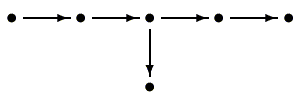In recent years I have concentrated on representations of quivers and preprojective algebras. A quiver is essentially the same thing as a directed graph, and a representation associates a vector space to each vertex and a linear map to each arrow. The subject was started by P. Gabriel in 1972, when he discovered that the quivers with only finitely many indecomposable representations are exactly the ADE Dynkin diagrams which occur in Lie theory (for example a quiver of type E6 is illustrated on the left). Quivers and their representations now appear in all sorts of areas of mathematics and physics, including representation theory, cluster algebras, geometry (algebraic, differential, symplectic), noncommutative geometry, quantum groups, string theory, and more.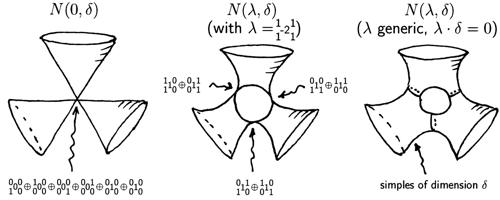The preprojective algebra associated to a quiver was invented by I. M. Gelfand and V. A. Ponomarev. Its modules are intimately related to representations of the quiver, but it is often the modules for the preprojective algebra which are of relevance in other parts of mathematics. There is beautiful geometry linked to the preprojective algebra, including Kleinian singularities and H. Nakajima's quiver varieties. (The illustration on the right shows the real-valued points of varieties associated to a quiver of extended Dynkin, that is, affine, type D4.)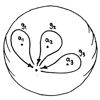There are also links between the preprojective algebra and the classification of differential equations on the Riemann sphere. They are used in work on the Deligne-Simpson problem, which concerns the existence of matrices in prescribed conjugacy classes whose product is the identity matrix, or whose sum is the zero matrix. (The picture on the left shows loops on the punctured Riemann sphere which generate its fundamental group. Consideration of the monodromy around such loops links the classification of differential equations on the Riemann sphere to the Deligne-Simpson problem.)

In earlier times I was interested in tame algebras, matrix problems, and infinite-dimensional modules. Finite dimensional associative algebras naturally divide into three classes: algebras finite representation type with only finitely many indecomposable modules, wild algebras for which the indecomposable modules are unclassifiable (in a suitable sense), and those on the boundary between these classes, the tame algebras. There are many interesting classes of tame algebras, and it is often a major problem to actually give the classification of the indecomposable modules.One way to study tame algebras is to convert the problem of classifying their modules into a matrix problem: putting a partitioned matrix into canonical form using not all elementary operations, but a subset defined by the partition. (The illustration on the right shows what an arbitrary matrix can be reduced to if you allow all row and column operations; it also shows an example of a partition of a matrix.) Using advanced methods based on this idea, Yu. A. Drozd proved his wonderful Tame and Wild Theorem showing that there is a wide gulf between the behaviour of tame and wild algebras. The same methods can be used to show that tame algebras are characterized by the behaviour of their infinite-dimensional modules. In fact, the behaviour of infinite-dimensional modules for tame algebras is extremely interesting, and not at all understood.

# Publications

1. Polycyclic-by-finite affine group schemes, Proc. London Math. Soc., 52 (1986), 495-516. (doi).
2. Locally finite representations of groups of finite p-rank, J. London Math. Soc., 34 (1986), 17-25. (doi).
3. (With P. H. Kropholler and P. A. Linnell) Torsion-free soluble groups and the zero-divisor conjecture, J. Pure and Appl. Algebra, 54 (1988), 181-196. (doi).
4. On tame algebras and bocses, Proc. London Math. Soc., 56 (1988), 451-483. (doi).
5. Functorial filtrations and the problem of an idempotent and a square zero matrix, J. London Math. Soc., 38 (1988), 385-402. (journal).
6. Functorial filtrations II: clans and the Gelfand problem, J. London Math. Soc., 40 (1989), 9-30. (doi).
7. Functorial filtrations III: semidihedral algebras, J. London Math. Soc., 40 (1989), 31-39. (doi).
8. Maps between representations of zero-relation algebras, J. Algebra, 126 (1989), 259-263. (doi).
9. (With L. Unger) Dimensions of Auslander-Reiten translates for representation-finite algebras, Comm. Algebra, 17 (1989), 837-842. (doi).
10. Matrix problems and Drozd's theorem, in 'Topics in Algebra', eds S. Balcerzyk et al., Banach Center publications, vol. 26 part 1 (PWN-Polish Scientific Publishers, Warsaw, 1990), 199-222. (pdf).
11. Regular modules for tame hereditary algebras, Proc. London Math. Soc., 62 (1991), 490-508. (doi).
12. Tame algebras and generic modules, Proc. London Math. Soc., 63 (1991), 241-265. (doi).
13. Lectures on representation theory and invariant theory, Ergänzungsreihe Sonderforschungsbereich 343 'Diskrete Strukturen in der Mathematik', 90-004, Bielefeld University, 1990, 74pp. (pdf).
14. Matrix reductions for artinian rings, and an application to rings of finite representation type, J. Algebra, 157 (1993), 1-25. (doi).
15. (With C. M. Ringel) Algebras whose Auslander-Reiten quiver has a large regular component, J. Algebra, 153 (1992), 494-516. (doi).
16. (With D. Happel and C. M. Ringel) A bypass of an arrow is sectional, Arch. Math. (Basel), 58 (1992), 525-528. (doi).
17. Modules of finite length over their endomorphism rings, in 'Representations of algebras and related topics', eds H. Tachikawa and S. Brenner, London Math. Soc. Lec. Note Series 168, (Cambridge University Press, 1992), 127-184. (pdf).
18. Additive functions on locally finitely presented Grothendieck categories, Comm. Algebra, 22 (1994), 1629-1639 (doi).
19. Locally finitely presented additive categories, Comm. Algebra, 22 (1994), 1641-1674. (doi).
20. Exceptional sequences of representations of quivers, in 'Representations of algebras', Proc. Ottawa 1992, eds V. Dlab and H. Lenzing, Canadian Math. Soc. Conf. Proc. 14 (Amer. Math. Soc., 1993), 117-124. (pdf).
21. (With O. Kerner) A functor between categories of regular modules for wild hereditary algebras, Math. Ann., 298 (1994), 481-487. (doi, GDZ).
22. (With D. J. Benson) A ramification formula for Poincaré series, and a hyperplane formula for modular invariants, Bull. London Math. Soc., 27 (1995), 435-440. (doi).
23. Subrepresentations of general representations of quivers, Bull. London Math. Soc., 28 (1996), 363-366. (doi).
24. (With R. Vila-Freyer) The structure of biserial algebras, J. London Math. Soc., 57 (1998), 41-54. (doi).
25. Rigid integral representations of quivers, in 'Representations of algebras', Proc. Cocoyoc 1994, eds R. Bautista et al., Canad. Math. Soc. Conf. Proc., 18 (Amer. Math. Soc., 1996), 155-163. (pdf).
26. Tameness of biserial algebras, Arch. Math. (Basel), 65 (1995), 399-407. (doi).
27. On homomorphisms from a fixed representation to a general representation of a quiver, Trans. Amer. Math. Soc., 348 (1996), 1909-1919. (doi).
28. (With M. P. Holland) Noncommutative deformations of Kleinian singularities, Duke Math. J., 92 (1998), 605-635. (doi).
29. Infinite-dimensional modules in the representation theory of finite-dimensional algebras, Canadian Math. Soc. Conf. Proc., 23 (1998), 29-54. (pdf).
30. Preprojective algebras, differential operators and a Conze embedding for deformations of Kleinian singularities, Comment. Math. Helv., 74 (1999), 548-574. (doi, pdf).
31. (With R. Bautista, T. Lei and Y. Zhang) On Homogeneous Exact Categories, J. Algebra, 230 (2000), 665-675. (doi).
32. On the exceptional fibres of Kleinian singularities, Amer. J. Math., 122 (2000), 1027-1037. (doi, pdf).
33. Geometry of the moment map for representations of quivers, Compositio Math., 126 (2001), 257-293. (doi, pdf).
34. Decomposition of Marsden-Weinstein reductions for representations of quivers, Compositio Math., 130 (2002), 225-239. (doi, math.AG/0007191).
35. (with Christof Geiß) Horn's problem and semi-stability for quiver representations, in 'Representations of Algebras, Vol 1', Proceedings of the Ninth International Conference, Beijing, August 21-September 1, 2000, eds. D. Happel and Y. B. Zhang (Beijing Normal University Press, 2002), 40-48. (pdf).
36. (with Jan Schröer) Irreducible components of varieties of modules, J. Reine Angew. Math. 553 (2002), 201-220. (doi, math.AG/0103100).
37. Normality of Marsden-Weinstein reductions for representations of quivers, Math. Ann. 325 (2003), 55-79. (doi, math.AG/0105247).
38. On matrices in prescribed conjugacy classes with no common invariant subspace and sum zero, Duke Math. J. 118 (2003), 339-352. (doi, math.RA/0103101).
39. (with Michel Van den Bergh) Absolutely indecomposable representations and Kac-Moody Lie algebras (with an appendix by Hiraku Nakajima), Invent. Math. 155 (2004), 537-559. (doi, pdf).
40. Indecomposable parabolic bundles and the existence of matrices in prescribed conjugacy class closures with product equal to the identity, Publ. Math. Inst. Hautes Etudes Sci. 100 (2004), 171-207. (doi, NUMDAM).
41. (with Peter Shaw) Multiplicative preprojective algebras, middle convolution and the Deligne-Simpson problem, Adv. Math. 201 (2006), 180-208. (doi).
42. (With Bernt Tore Jensen) A note on sub-bundles of vector bundles, Glasgow Math. J. 48 (2006), 459-462. (doi, pdf).
43. Quiver algebras, weighted projective lines, and the Deligne-Simpson problem, in: 'Proceedings of the International Congress of Mathematicians', vol. 2, Madrid 2006, eds M. Sanz-Solé et al. (European Mathematical Society, January 2007), 117-129. (pdf).
44. (With Pavel Etingof and Victor Ginzburg) Noncommutative Geometry and Quiver algebras, Adv. Math. 209 (2007), 274-336. (doi).
45. General sheaves over weighted projective lines, Colloq. Math. 113 (2008), 119-149. (doi, EUDML).
46. Kac's Theorem for weighted projective lines, Journal of the European Mathematical Society, 12 (2010), 1331-1345. (doi, math.AG/0512078).
47. Connections for weighted projective lines, J. Pure Appl. Algebra, 215 (2011), 35-43. (doi).
48. Poisson structures on moduli spaces of representations, J. Algebra 325 (2011), 205-215 (doi).
49. Monodromy for systems of vector bundles and multiplicative preprojective algebras, Bulletin of the London Mathematical Society 45 (2013), 309-317. (doi)
50. Kac's Theorem for equipped graphs and for maximal rank representations, Proceedings of the Edinburgh Mathematical Society 56 (2013), 443-447. (doi, pdf).
51. Decomposition of pointwise finite-dimensional persistence modules, Journal of Algebra and Its Applications Vol. 14, No. 5 (2015), 1550066 (8 pages). (doi, arXiv:1210.0819 [math.RT]).
52. (With Frédéric Chazal and Vin de Silva) The observable structure of persistence modules, Homology, Homotopy and Applications 18 (2016), 247-265. (doi, pdf)
53. (With Julia Sauter) On quiver Grassmannians and orbit closures for representation-finite algebras, Mathematische Zeitschrift 285 (2017), 367–395. (open access doi)
54. Classification of modules for infinite-dimensional string algebras, Transactions of the American Mathematical Society, 370 (2018), 3289-3313. (doi, pdf)
55. Representations of equipped graphs: Auslander-Reiten theory, in: Proceedings of the 50th Symposium on Ring Theory and Representation Theory. Held at the University of Yamanashi, October 7–10, 2017. Edited by Katsunori Sanada. Symposium on Ring Theory and Representation Theory Organizing Committee, Yamanashi, 2018. (pdf, entire proceedings)
56. (With Raphael Bennett-Tennenhaus) Σ-pure-injective modules for string algebras and linear relations, Journal of Algebra 513 (2018), 177-189. (doi, pdf)
57. (With Andrew Hubery) A new approach to simple modules for preprojective algebras, Algebras and Representation Theory 23 (2020), 1849-1860. (doi, arxiv:1803.09482 [math.RT])
58. (With Magnus Bakke Botnan) Decomposition of persistence modules, Proceedings of the American Mathematical Society 148 (2020), 4581-4596. (doi, arxiv:1811.08946 [math.RT])
59. (With Biao Ma, Baptiste Rognerud and Julia Sauter) Combinatorics of faithfully balanced modules, Journal of Combinatorial Theory. Series A. 182 (2021), 105472, 45pp. (doi, arXiv:1905.00613 [math.RT])
60. (With Yuta Kimura) On deformed preprojective algebras, Journal of Pure and Applied Algebra 226 (2022), 107130, 22pp. (doi, arXiv:2108.00795)

# Preprints

ArXiv author page

• (With Raphael Bennett-Tennenhaus) Semilinear clannish algebras, arXiv:2204.12138
• Rigid integral representations of quivers over arbitrary commutative rings, arXiv:2301.04941

# Knitting

An applet written by Jan Geuenich (at my request) to compute Auslander-Reiten quivers by the knitting algorithm. It either computes the preprojective component, or the entire AR quiver if the algebra is of finite representation type and representation-directed.

Call it without an argument to do a random example:
https://www.math.uni-bielefeld.de/~wcrawley/knitting/
The simple modules are labelled by digits or letters. Place the cursor over a vertex to display the dimension vector of the corresponding indecomposable module, as a list of simples with appropriate multiplicities.

In general one needs to specify the labels of the simple modules and the dimension vectors of the indecomposable direct summands of the radicals of their projective covers. For example the path algebra of quiverhas simples 1,2,3,4 and the radicals of the projective covers P and P are zero, the radical of P is a direct sum of simples 1 and 2 and the radical of P is indecomposable of dimension vector 123. Compute the AR quiver with
https://www.math.uni-bielefeld.de/~wcrawley/knitting/?projectives=1:,2:,3:1+2,4:123

The commutative square algebra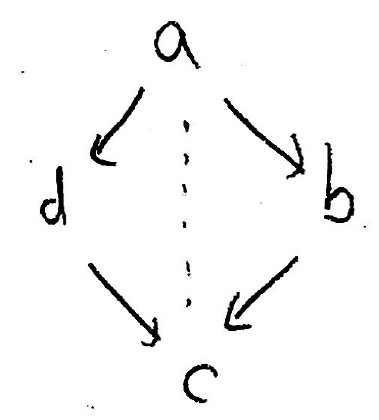has simples a,b,c,d and rad P[a] is indecomposable of dimension vector bcd, rad P[b] has dimension vector c, rad P[c] is zero and rad P[d] has dimension vector c. Compute the AR quiver with
https://www.math.uni-bielefeld.de/~wcrawley/knitting/?projectives=a:bcd,b:c,c:,d:c

The path algebra of the quiver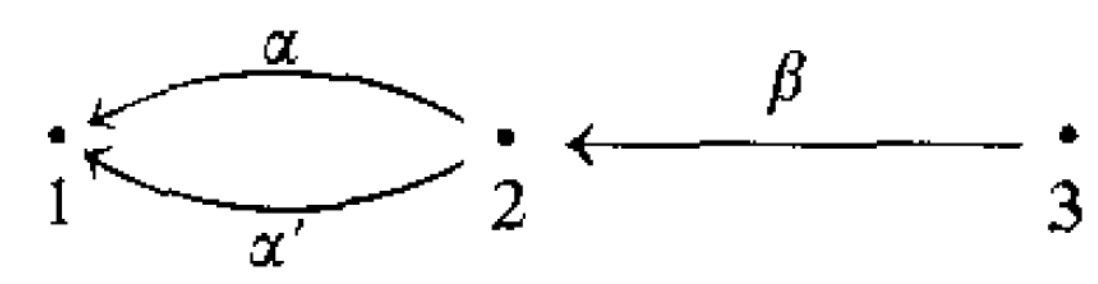has preprojective component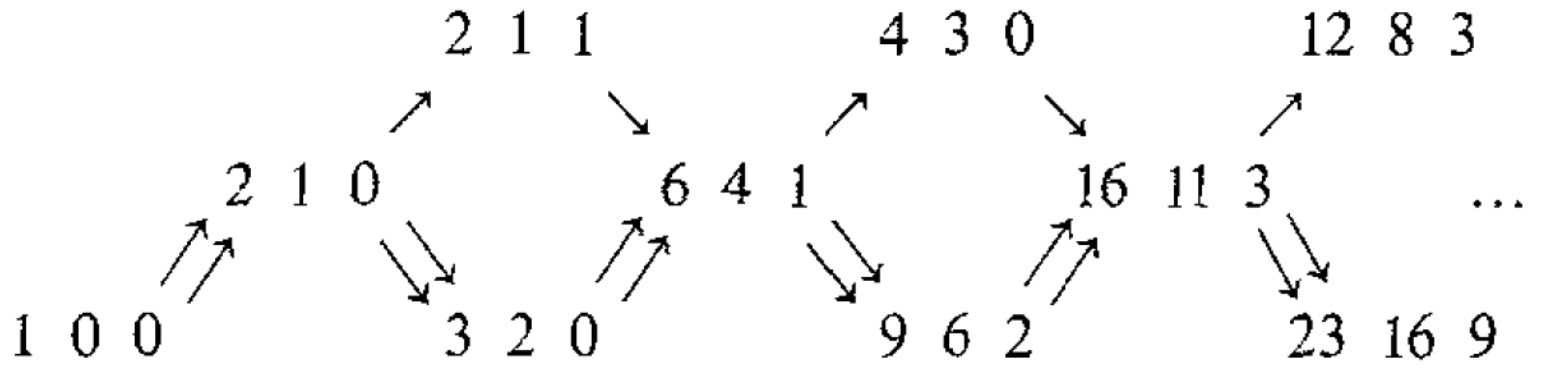displayed in C. M. Ringel, Finite dimensional hereditary algebras of wild representation type, Mathematische Zeitschrift 161 (1978), 235-255. Now rad P is zero, rad P has two indecomposable summands of dimension vector 1 and rad P is indecomposable of dimension vector 122, so the preprojective component is computed with:
https://www.math.uni-bielefeld.de/~wcrawley/knitting/?projectives=1:,2:1+1,3:112
(Find the misprint.)

You can drag the projective vertices to new positions, or specify them with the option "positions=". Another example, taken from section 6.6 of P. Gabriel, Auslander-Reiten sequences and representation-finite algebras, in "Representation theory, I" (Proc. Workshop, Carleton Univ., Ottawa, Ont., 1979), pp. 1–71, Lecture Notes in Math., 831, Springer, Berlin, 1980. The algebra with quiver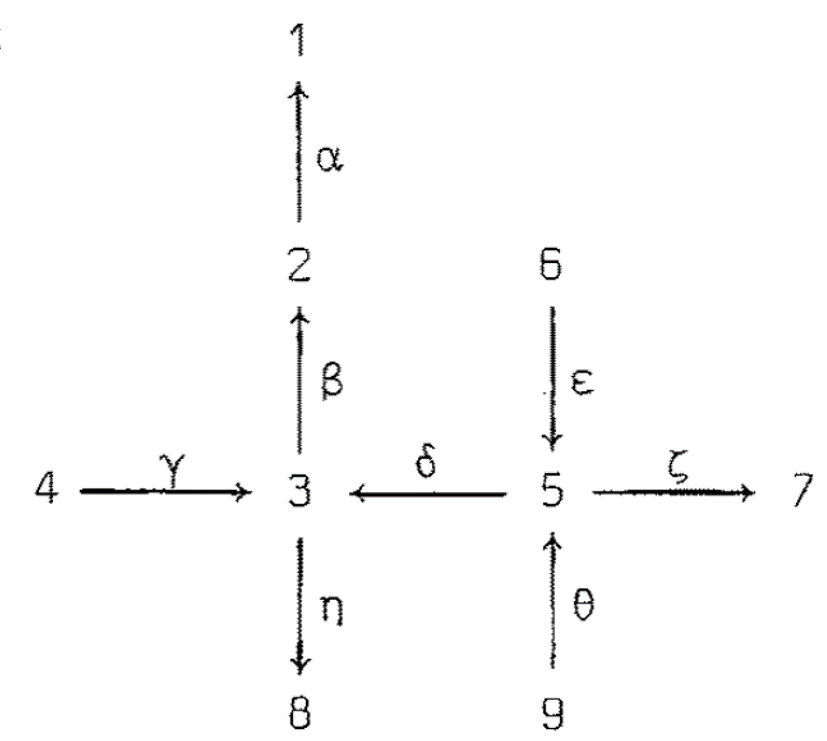and relations βγ = βδε = ζε = ηδθ = 0 has AR quiver
https://www.math.uni-bielefeld.de/~wcrawley/knitting/?projectives=1:,2:1,3:12+8,4:38,5:1238+7,6:358,7:,8:,9:12357&positions=1:3,2:4,3:5,4:2,5:6,6:0,7:7,8:5.2,9:1
or
https://www.math.uni-bielefeld.de/~wcrawley/knitting/?projectives=1:,2:1,3:12+8,4:38,5:1238+7,6:358,7:,8:,9:12357&positions=1:(-50|0),2:(0|0),3:(50|0),4:(-100|1),5:(100|0),6:(-200|0),7:(150|0),8:(60|0),9:(-150|0)

You can draw the AR quiver vertically with the option "orientation=vertical".
https://www.math.uni-bielefeld.de/~wcrawley/knitting/?projectives=1:,2:1,3:12+8,4:38,5:1238+7,6:358,7:,8:,9:12357&positions=1:(-50|0),2:(0|0),3:(50|0),4:(-100|1),5:(100|0),6:(-200|0),7:(150|0),8:(60|0),9:(-150|0)&orientation=vertical

Note that for the knitting procedure to be valid, you should check beforehand that all indecomposable summands of the radicals are the unique indecomposable modules of their dimension vectors. This is in any case true for modules in preprojective components. It is assumed that the base field is algebraically closed, or more generally that every simple module has endomorphism algebra equal to the base field (equivalently that the algebra is given by a quiver with admissible relations).

# Archived materials

• Lectures on representation theory and invariant theory (pdf). A graduate course given in 1989/90 at Bielefeld University. This is an introduction to the representation theory of the symmetric and general linear groups (in characteristic zero), and to classical invariant theory. List of corrections.
• Lectures on representations of quivers (pdf | scanned pdf - includes one extra diagram). A graduate course given in 1992 at Oxford University. This is an introduction to the representation theory of quivers, and in particular the representation theory of extended Dynkin quivers. List of corrections.
• More lectures on representations of quivers (scanned pdf). Another graduate course from 1992 at Oxford University. More about representations of quivers, including Auslander-Reiten theory, results of Kerner and of Schofield.
• Geometry of representations of algebras (pdf). A graduate course given in 1993 at Oxford University. This is a survey of how algebraic geometry has been used to study representations of algebras (and quivers in particular).
• Cohomology and central simple algebras (pdf). An MSc course given in 1996 at Leeds University. An introduction to homological algebra and applications to central simple algebras.
• Representations of quivers, preprojective algebras and deformations of quotient singularities (pdf). Lectures from a DMV Seminar in May 1999 on "Quantizations of Kleinian singularities" organized by R. Buchweitz, P. Slodowy and myself at Oberwolfach. Here is the group photo from the meeting. (There are mistakes in Lemma 4.5 and the proof of Theorem 5.9. The first of these has been sorted out independently by P. Etingof and V. Ginzburg in math.AG/0011114. For the second, the proof elsewhere in the literature is correct.)
• The website from a Summer School on "Geometry of Quiver-representations and Preprojective Algebras" (Isle of Thorns/UK, September 10 - 17, 2000)
• An unfinished and abandoned paper on generic deformed preprojective algebras (pdf), dating from the late 1990s, and mentioned by P. Etingof and E. Rains in math.RT/0503393.
• The website for a conference in honour of John McConnell and Chris Robson (Leeds, May 5-6, 2006)
• The slides of a talk in Glasgow in November 2014 on "Two applications of the functorial filtration method".
• Notes for a working seminar on "Sylvester rank functions for rings and universal localization" in June 2021.